Publicité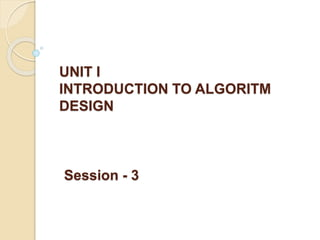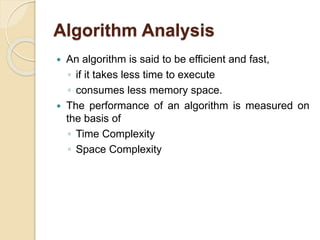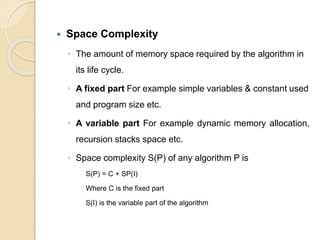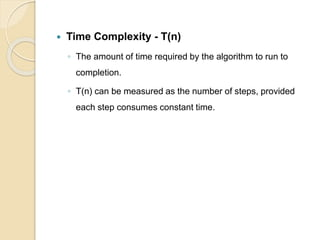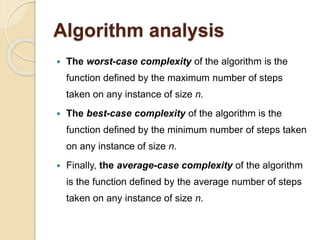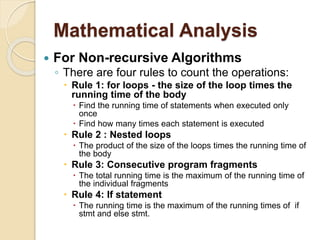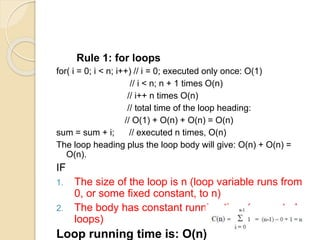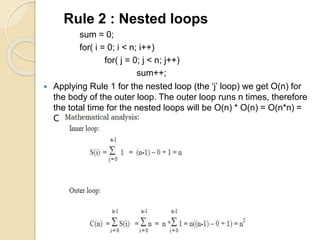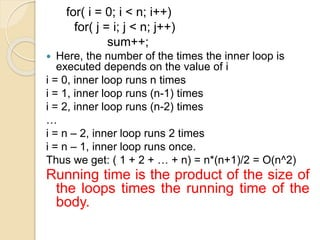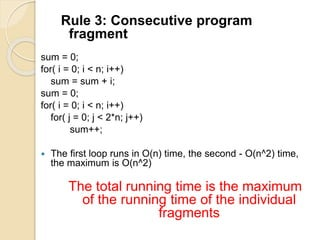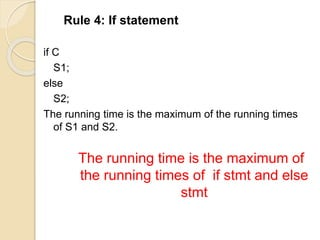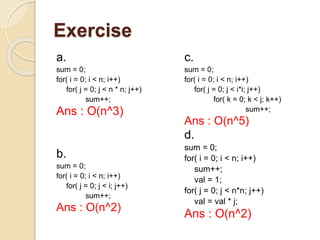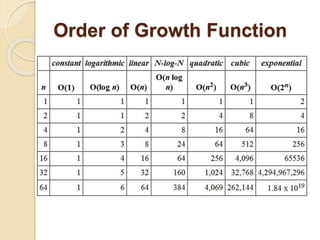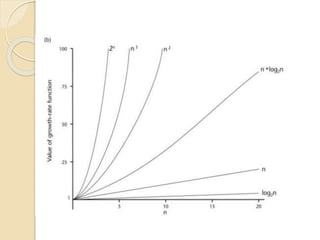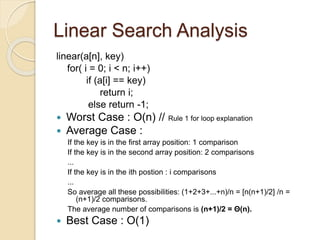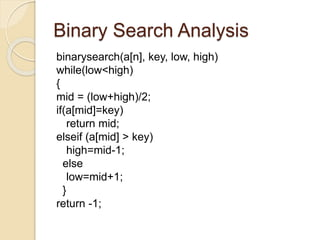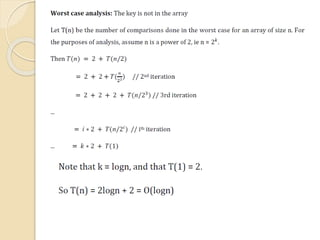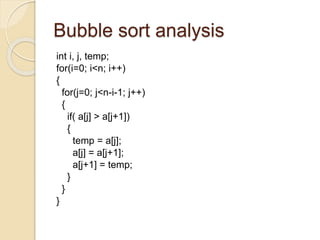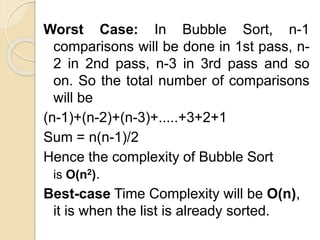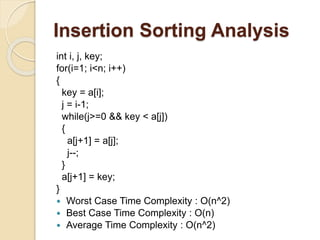1 sur 20
Publicité

### UNIT I- Session 3.pptx

1. UNIT I INTRODUCTION TO ALGORITM DESIGN Session - 3
2. Algorithm Analysis  An algorithm is said to be efficient and fast, ◦ if it takes less time to execute ◦ consumes less memory space.  The performance of an algorithm is measured on the basis of ◦ Time Complexity ◦ Space Complexity
3.  Space Complexity ◦ The amount of memory space required by the algorithm in its life cycle. ◦ A fixed part For example simple variables & constant used and program size etc. ◦ A variable part For example dynamic memory allocation, recursion stacks space etc. ◦ Space complexity S(P) of any algorithm P is S(P) = C + SP(I) Where C is the fixed part S(I) is the variable part of the algorithm
4.  Time Complexity - T(n) ◦ The amount of time required by the algorithm to run to completion. ◦ T(n) can be measured as the number of steps, provided each step consumes constant time.
5. Algorithm analysis  The worst-case complexity of the algorithm is the function defined by the maximum number of steps taken on any instance of size n.  The best-case complexity of the algorithm is the function defined by the minimum number of steps taken on any instance of size n.  Finally, the average-case complexity of the algorithm is the function defined by the average number of steps taken on any instance of size n.
6. Mathematical Analysis  For Non-recursive Algorithms ◦ There are four rules to count the operations:  Rule 1: for loops - the size of the loop times the running time of the body  Find the running time of statements when executed only once  Find how many times each statement is executed  Rule 2 : Nested loops  The product of the size of the loops times the running time of the body  Rule 3: Consecutive program fragments  The total running time is the maximum of the running time of the individual fragments  Rule 4: If statement  The running time is the maximum of the running times of if stmt and else stmt.
7. Rule 1: for loops for( i = 0; i < n; i++) // i = 0; executed only once: O(1) // i < n; n + 1 times O(n) // i++ n times O(n) // total time of the loop heading: // O(1) + O(n) + O(n) = O(n) sum = sum + i; // executed n times, O(n) The loop heading plus the loop body will give: O(n) + O(n) = O(n). IF 1. The size of the loop is n (loop variable runs from 0, or some fixed constant, to n) 2. The body has constant running time (no nested loops) Loop running time is: O(n)
8. Rule 2 : Nested loops sum = 0; for( i = 0; i < n; i++) for( j = 0; j < n; j++) sum++;  Applying Rule 1 for the nested loop (the ‘j’ loop) we get O(n) for the body of the outer loop. The outer loop runs n times, therefore the total time for the nested loops will be O(n) * O(n) = O(n*n) = O(n^2)
9. for( i = 0; i < n; i++) for( j = i; j < n; j++) sum++;  Here, the number of the times the inner loop is executed depends on the value of i i = 0, inner loop runs n times i = 1, inner loop runs (n-1) times i = 2, inner loop runs (n-2) times … i = n – 2, inner loop runs 2 times i = n – 1, inner loop runs once. Thus we get: ( 1 + 2 + … + n) = n*(n+1)/2 = O(n^2) Running time is the product of the size of the loops times the running time of the body.
10. Rule 3: Consecutive program fragment sum = 0; for( i = 0; i < n; i++) sum = sum + i; sum = 0; for( i = 0; i < n; i++) for( j = 0; j < 2*n; j++) sum++;  The first loop runs in O(n) time, the second - O(n^2) time, the maximum is O(n^2) The total running time is the maximum of the running time of the individual fragments
11. Rule 4: If statement if C S1; else S2; The running time is the maximum of the running times of S1 and S2. The running time is the maximum of the running times of if stmt and else stmt
12. Exercise a. sum = 0; for( i = 0; i < n; i++) for( j = 0; j < n * n; j++) sum++; Ans : O(n^3) b. sum = 0; for( i = 0; i < n; i++) for( j = 0; j < i; j++) sum++; Ans : O(n^2) c. sum = 0; for( i = 0; i < n; i++) for( j = 0; j < i*i; j++) for( k = 0; k < j; k++) sum++; Ans : O(n^5) d. sum = 0; for( i = 0; i < n; i++) sum++; val = 1; for( j = 0; j < n*n; j++) val = val * j; Ans : O(n^2)
13. Order of Growth Function
14. Linear Search Analysis linear(a[n], key) for( i = 0; i < n; i++) if (a[i] == key) return i; else return -1;  Worst Case : O(n) // Rule 1 for loop explanation  Average Case : If the key is in the first array position: 1 comparison If the key is in the second array position: 2 comparisons ... If the key is in the ith postion : i comparisons ... So average all these possibilities: (1+2+3+...+n)/n = [n(n+1)/2] /n = (n+1)/2 comparisons. The average number of comparisons is (n+1)/2 = Θ(n).  Best Case : O(1)
15. Binary Search Analysis binarysearch(a[n], key, low, high) while(low<high) { mid = (low+high)/2; if(a[mid]=key) return mid; elseif (a[mid] > key) high=mid-1; else low=mid+1; } return -1;
16. Bubble sort analysis int i, j, temp; for(i=0; i<n; i++) { for(j=0; j<n-i-1; j++) { if( a[j] > a[j+1]) { temp = a[j]; a[j] = a[j+1]; a[j+1] = temp; } } }
17. Worst Case: In Bubble Sort, n-1 comparisons will be done in 1st pass, n- 2 in 2nd pass, n-3 in 3rd pass and so on. So the total number of comparisons will be (n-1)+(n-2)+(n-3)+.....+3+2+1 Sum = n(n-1)/2 Hence the complexity of Bubble Sort is O(n2). Best-case Time Complexity will be O(n), it is when the list is already sorted.
18. Insertion Sorting Analysis int i, j, key; for(i=1; i<n; i++) { key = a[i]; j = i-1; while(j>=0 && key < a[j]) { a[j+1] = a[j]; j--; } a[j+1] = key; }  Worst Case Time Complexity : O(n^2)  Best Case Time Complexity : O(n)  Average Time Complexity : O(n^2)
Publicité